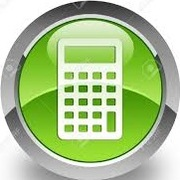# Learn Differential Equations

Study Differential Equations using smart web & mobile flashcards created by top students, teachers, and professors. Prep for a quiz or learn for fun!

### Top Differential Equations Flashcards Ranked by Quality

• MATH2375 Linear Differential EquationsShow Class
• MATH2391 Non-Linear Differential EquationsShow Class
• Differential EquationsShow Class
• Differential EquationsShow Class
• Differential EquationsShow Class
• Differential EquationsShow Class
• Differential EquationsShow Class
• CalculusShow Class
• ► CalculusShow Class
• B) CalculusShow Class
• Z calculasShow Class
• Bryx_MathShow Class
• A-Level Further MathsShow Class
• Year 1Show Class
• CalculusShow Class
• A Level - MathsShow Class
• MathShow Class
• CfE Higer Maths FormulasShow Class
• Z - AP CalculusShow Class
• A2 Maths C4Show Class
• MathsShow Class
• USMLE Step IShow Class
• Matematikk 1Show Class
• A2 MathsShow ClassShow Class
• Calculus 1Show Class
• MathematicsShow Class
• MathsShow Class
• PHY250 - Electromagnetism to QuantumShow Class
• Biology OCR BShow Class
• Numerical - MichaelmasShow Class
• Mathematics 144Show Class
• AP Calculus ABShow Class
• Stochastic Processesand FinanceShow Class
• MATH 138Show Class
• PHAS0009Show Class
• Maths—C1Show Class
• Yr 13 Further Maths PureShow Class
• FP2Show Class
• HTM 381 Revenue ManagementShow Class
• PHAS0025Show Class
• Maths PureShow Class
• CalculusShow Class
• Maths C4Show Class
• Maths (2015 modules)Show Class
• C4 MathsShow Class
• Pure Maths Year 1Show Class
• MA1003Show Class
• MCSShow Class
• 1 MathsShow Class